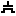#Transistor

 Library: Wiring Introduced: 2.7.0 Appearance:## Behavior

A transistor has two inputs, called gate and source, and one output, called drain. When diagrammed, the source input and drain output are drawn connected by a plate; Logisim draws an arrowhead to indicate the direction of flow from input to output. The gate input is drawn connected to a plate that is parallel to the plate connecting source to drain. Logisim supports two types of transistors, with slightly different behaviors described below; the P-type transistor is indicated by a circle connecting the gate input to its plate, while the N-type transistor has no such circle.

Depending on the value found at gate, the value at source may be transmitted to drain; or there may be no connection from source, so drain is left floating. The determination of transmitting or disconnecting depends on the type of transistor: A P-type transistor (indicated by a circle on the gate line) transmits when gate is 0, while an N-type transistor (which has no such circle) transmits when gate is 1. The behavior is summarized by the following tables.

P-type gate 0 10 Z X 1 Z X Z Z Z X Z X

N-type gate 0 1Z 0 X Z 1 X Z Z Z Z X X

Or in summarized form:

P-typegatedrain
0source
1Z
X/ZX*

N-typegatedrain
0Z
1source
X/ZX*

* If source is Z, drain is Z; otherwise drain is X.

If the Data Bits attribute is more than 1, the gate input is still a single bit, but its value is applied simultaneously to each of the source input's bits.

An N-type transistor behaves very similarly to a Controlled Buffer. The primary difference is that a transistor is meant for more basic circuit designs.

## Pins (assuming component faces east, gate line top/left)

West edge (input, bit width matches Data Bits attribute)
The component's source input that will transmit to the output if triggered by the gate input.
North edge (input, bit width 1)
The component's gate input. For P-type transistors, the transistor will transmit if the gate value is 0; for N-type transistors, this will trigger the transistor if the gate value is 1.
East edge (output, bit width matches Data Bits attribute)
The component's output, which will match the source input if indicated by the gate input, or will be floating if the gate input is the negation of what indicates negation. If gate is floating or an error value, then the output will be an error value.

## Attributes

When the component is selected or being added, Alt-0 through Alt-9 alter its Data Bits attribute and the arrow keys alter its Facing attribute.

Type
Determines whether the transistor is P-type or N-type.
Facing
The direction of the component (its output relative to its input).
Gate Location
The location of the gate input.
Data Bits
The bit width of the component's inputs and outputs.

None.

## Text Tool Behavior

None.

Back to Library Reference